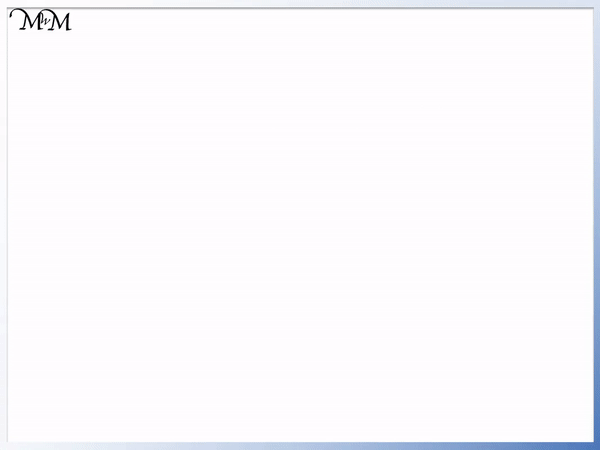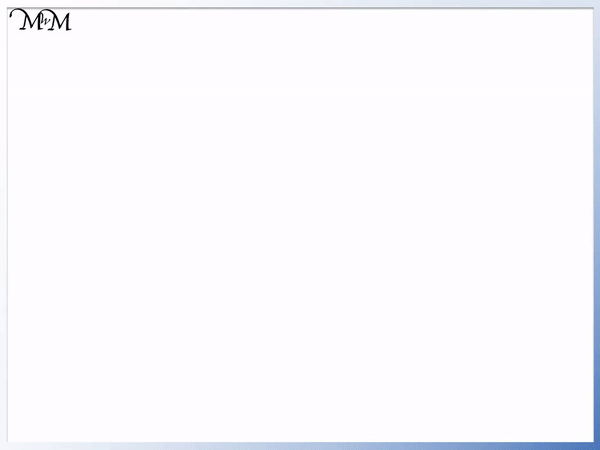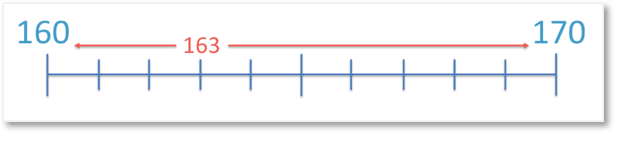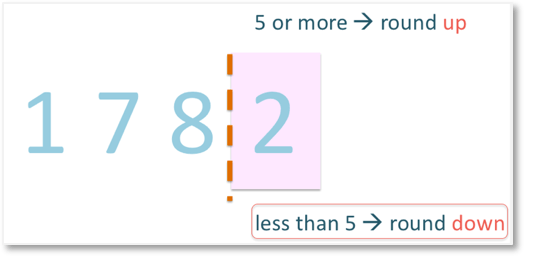# Rounding to the Nearest Ten

Rounding to the Nearest Ten#### Rounding to the Nearest Ten Summary• Rounding to the nearest ten means to find the nearest number in the ten times table.
• 163 is nearer to 160 than it is to 170.
• 166 is nearer to 170 than it is to 160.
• 165 is exactly in between 160 and 170 but we still decide to round it up to 170.
• We always look at the digit in the units column to help us decide whether to round up or round down.

If a whole number ends in: 0, 1, 2, 3 or 4, we round down.

If a whole number end in: 5, 6, 7, 8 or 9, we round up.• We want to round 1782 to the nearest ten.
• We look at the units column to help us decide whether to round down to 1780 or up to 1790.
• If the digit in the units column is 5 or more, we round up. Otherwise we round down.
• 2 is less than 5 so we will round down.
• 1782 rounded to the nearest ten is 1780.# Rules for Rounding Off Numbers to the Nearest 10

Rounding Numbers (or rounding off numbers) to the nearest ten means that we write down the nearest number in the ten times table to our original number.

We actually replace the original number with a less-accurate but often easier-to-use number.

When we round to the nearest ten we will always replace our original number with the nearest number in the ten times table and numbers in the ten times table can be easier to work with in some calculations.

For example, round 163 to the nearest 10.The number line shows that the two nearest tens to 163 are 160 and 170.

To decide whether we round down to 160 or up to 170, we look at which number is the nearest.

163 already contains 16 tens (160) plus 3 more units.

Notice that our choice of values to round off to will be either to keep the 160 that we have (rounding down) or to go up to the next ten along (rounding up).163 is nearer to 160 than to 170.

Therefore, we round 163 down to 160.

Here’s another example of rounding off:

Round 166 to the nearest 10.The two nearest tens to 166 are 160 and 170.

166 already contains 16 tens (160) plus 6 more units.

Notice that our choice of values to round off to are the same as in the previous example. We can keep the 160 we have (rounding down) or we can go up to the next ten along (rounding up).

To decide whether we round down to 160 or up to 170, we look at which number is the nearest to 166 on our number line.166 is nearer to 170 than to 160. Therefore, we round 166 up to 170.

Here’s our next rounding off example with a number ending in 5:

We are asked to round 165 to the nearest 10.The two nearest tens to 165 are 160 and 170.

To decide whether we round down to 160 or up to 170, we look at which number is the nearest.165 is the same distance to 160 as it is to 170. So, does 5 round up or down?

We round a whole number ending in 5 or more up to the nearest ten.

This is because if any other digits were to follow the 5, they would make the number slightly closer to the larger number than the smaller number.

For example, if the number was 165.1, it would be slightly closer to 170 than to 160. By always rounding a number with 5 in the units column up, we keep a consistent rounding method even when decimal numbers are involved.

From the examples that we have looked at, we can create a general rule for rounding whole numbers to the nearest 10:

If the digit in the units column is less than 5, we round down.

If the digit in the units column is 5 or more, we round up.

Another way to think of this rounding rule is:

If the digit in the units column is 0, 1, 2, 3 or 4, we round down.

If the digit in the units column is 5, 6, 7, 8 or 9, we round up.

Rounding Example 1:

Round 145 to the nearest ten.The two nearest tens to 145 are 140 and 150.

Remember, the two choices of tens that we could round to is between the amount of tens we already have: 140, or the next ten along: 150.

To decide whether we round up or down, we only look at the units column.

Because we have 5, we round up to 150.

Rounding Example 2:Round 1782 to the nearest ten.

The two nearest tens are 1780 and 1790.

To decide whether we round up or down, we look at the units column. 2 is less than 5, so we round down to 1780.

Rounding Example 3:

Round 297 to the nearest ten.The two nearest tens are 290 and 300.

To decide whether we round up or down, we look at the units column.

7 is greater than 5, so we round up to 300.

This rounding rule for looking at the units column will work with all numbers being rounded to the nearest ten.Now try our lesson on Rounding Decimals to the Nearest Whole Number where we extend our rounding rules to decimal numbers to allow us to find the closest whole number.